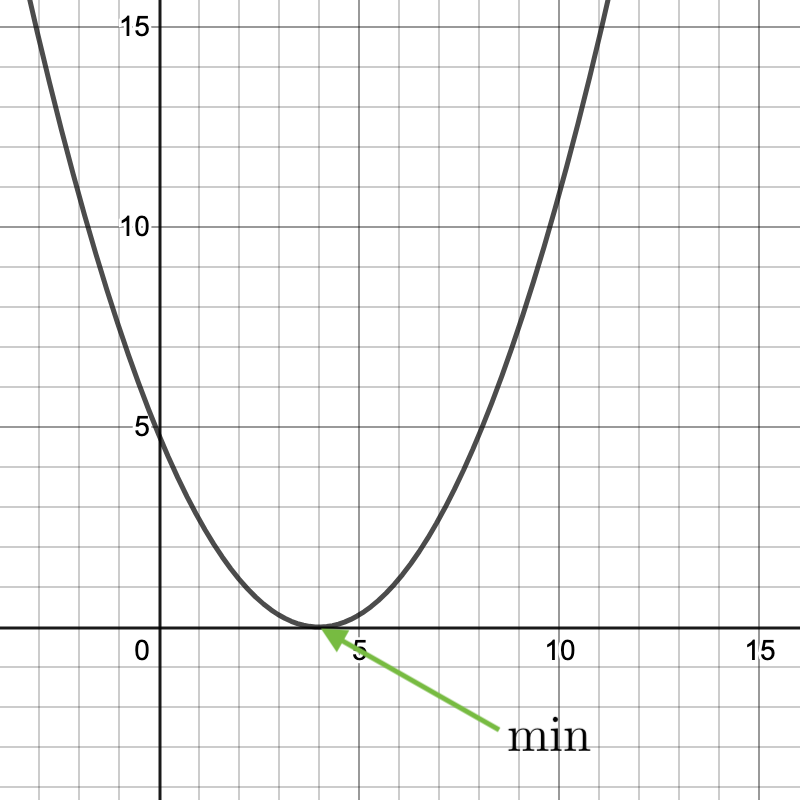# Gradient descent for univariate linear regression

Gradient descent is used for many aspects of machine learning. One area in which it is used a ton is in optimizing artificial neural networks. I will explain the concept of how this works once you understand the basic gradient descent operations.

Gradient descent can also be used to minimize arbitrary functions such as more general ones like cost functions with $$n$$ number of parameters: $$J(\theta _0, \theta _1, \theta _2, \theta _3, … , \theta _n)$$.

Please keep in mind that gradient descent is NOT HARD. Especially understanding how it minimizes functions is super easy to get. So, if you don’t understand a concept right away, keep on trying to see it from different angles and I’m sure it’ll click.

So, without further ado let me give you the general outline for minimizing a function using G.D.:

1. Start with some value for $$\theta _0$$and $$\theta _1$$
2. Keep changing $$\theta _0$$and $$\theta _1$$to reduce $$(\theta _0, \theta _1)$$until we hopefully find a minimum

Note: The assignment operator in maths looks like this: “$$:=$$”. It can also be used to reassign variable values. The normal “$$=$$” is a truth assertion.

Here are some variable to know before I get into the algorithm:

$$\alpha=$$”alpha” is the learning rate. It is how much we change the parameter values by each update step. It usually is a constant. Ex: If $$\alpha=10$$whatever value the algorithm gives to update the parameter value by, it is multiplied by a factor of ten.

$$j=$$it is a subscript to $$\theta$$to represent a which parameter we are dealing with. It is a generic form of saying something like $$theta _0. And a refresher on the cost function: J(\theta _0,\theta _1)=\frac{1}{2m} \sum _{i=1} ^m (h _\theta (x^i) - y^i)^2 Here is the actual algorithm: repeat \hspace{2mm} until \hspace{2mm}convergence \hspace{4mm} \{ \\ \hspace{10mm} \theta _j := \theta _j \hspace{1mm}- \hspace{1mm}\alpha \frac{\partial J(\theta _ 0, \theta _ 1)}{\partial \theta _j }\\ \} \hspace{67mm} This is repeated simultaneously for \(\theta _0$$and $$\theta _1$$. A.K.A this is repeated simultaneously for the $$j$$values of $$0,1$$.

Programmatically, you will have to create temporary variables to be able to simulate a simultaneous update.

Correct Simultaneous Update:

$temp0 := \theta _0 \hspace{1mm}- \hspace{1mm}\alpha \frac{\partial J(\theta _0, \theta _1)}{\partial \theta _0 } \\ temp1 := \theta _1 \hspace{1mm}- \hspace{1mm}\alpha \frac{\partial J(\theta _0, \theta _1)}{\partial \theta _1 } \\ \\ \\ \\ \theta _0 := temp0 \\ \theta _1 := temp1$

INcorrect Simultaneous Update:

$temp0 := \theta _0 \hspace{1mm}- \hspace{1mm}\alpha \frac{\partial J(\theta _0, \theta _1)}{\partial \theta _0 } \\ \theta _0 := temp0 \\ temp1 := \theta _1 \hspace{1mm}- \hspace{1mm}\alpha \frac{\partial J(\theta _0, \theta _1)}{\partial \theta _1 } \\ \theta _1 := temp1$

The error here occurs because $$\theta _0$$is updated before $$\theta _1$$. That means that $$\theta _1$$is using the newly updated value of $$\theta _0$$to calculate the partial derivative with respects to $$\theta _1$$which is NOT what we want to happen.

## Example Using an Easier Function

Let’s say that our task was to minimize some arbitrary cost function with one parameter $$(\theta _1)$$where $$theta _1 \in ℝ$$. The graph of the function $$(\theta _1)$$(if it is a squared error cost function) might look something like this:What we want to find is the value of $$\theta$$ that makes the $$y$$component of this graph to be as low as possible – in this case, 0. Just looking at the graph, we can see that that number is 4. But, in more complex cost functions that deal with hundreds of parameters/dimensions, simply graphing the cost function will not yield an easy-to-see answer.

In terms of gradient descent, we start by choosing a random value for $$\theta _1$$(in more advanced implementations, there are more clever ways to choose a starting value, but whatever). We then find the slope of the chosen point on the graph and subtract it from $$\theta _1’s current value. To find the slope we find the derivative of the cost function with respect to \(\theta _1$$.

Note: If you don’t know calc already, THAT’S TOTALLY OKAY! I didn’t either when I started learning about this subject. I taught all I needed to know to myself. I would highly highly recommend 3Blue1Brown’s “The Essence of Calculus” as a starting point. It has amazing animations and fantastic descriptions of everything you would need to know. If you want to just keep trudging along, though, just note that the derivative of a function allows you to find the slope of any point along that original function.

Okay, back to it.

Here is the expression for updating our parameter:

$\theta _1 := \theta _1 - \alpha \frac{d J(\theta _1)}{d\theta _1}$

Next:

• Example using easier function, J(theta 1)